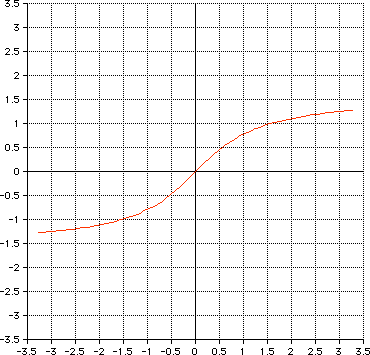drive.web Function Block Engine Math Library

General

The Math Library includes algebraic and transcendental functions. These are implemented for the basic drive.web data type of signed 16-bit fixed-point numbers. This data type imposes domain and codomain limitations which must be considered by the user. Each function along with its domain and codomain limitations are described below.

Square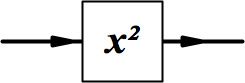Domain -3.2767 to +3.2767
-1.8101 to +1.8101 without hitting output clamps
Codomain 0 to +3.2767
Graph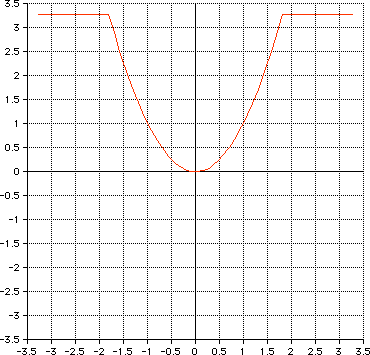Square Root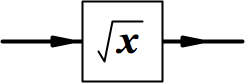Domain -3.2767 to +3.2767
(negative inputs are treated as positive inputs)
Codomain 0 to +1.8101
Graph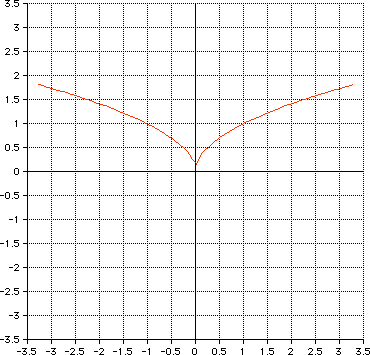Cube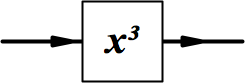Domain -3.2767 to +3.2767
-1.4853 to +1.4853 without hitting output clamps
Codomain -3.2767 to +3.2767
Graph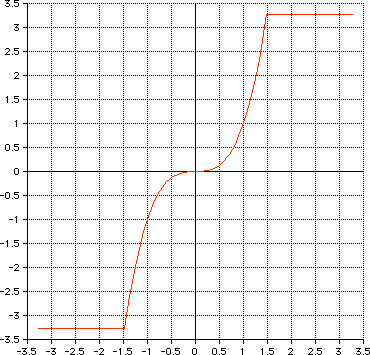Cube Root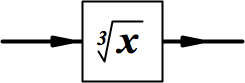Domain -3.2767 to +3.2767
Codomain -1.4853 to +1.4853
Graph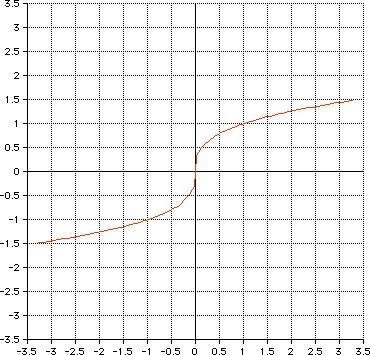Reciprocal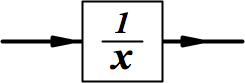Domain -3.2767 to +3.2767
-3.2767 to -0.3052 and +0.3052 to +3.2767 without
hitting output clamps
Codomain -3.2767 to -0.3052 and +0.3052 to +3.2767
Graph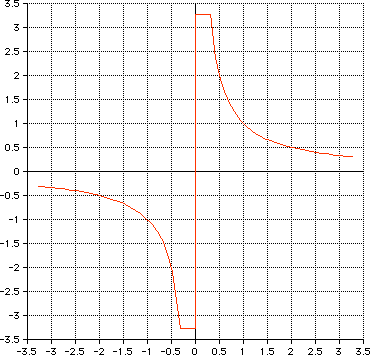Exponential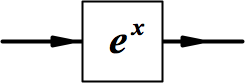Domain -3.2767 to +3.2767
-3.2767 to +1.1868 without hitting output clamps
Codomain +0.0378 to +3.2767
Graph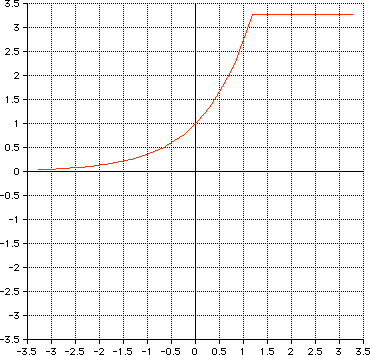Logarithm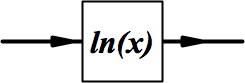Domain -3.2767 to +3.2767
(negative inputs are treated as positive inputs)
+0.0378 to +3.2767 without hitting output clamps
Codomain -3.2767 to +1.1868
Graph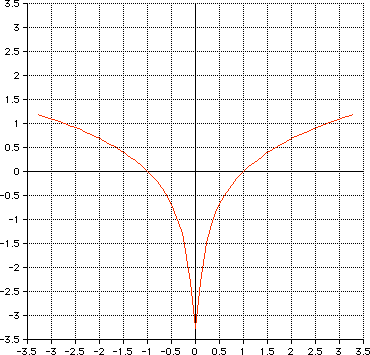Sine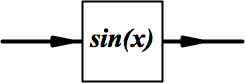Codomain -1 to +1
Graph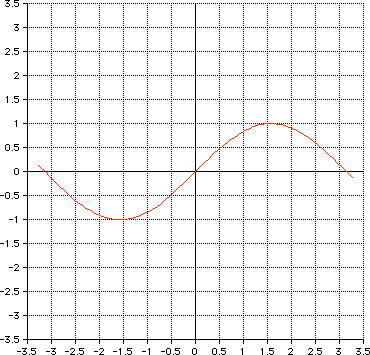Cosine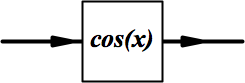Codomain -1 to +1
Graph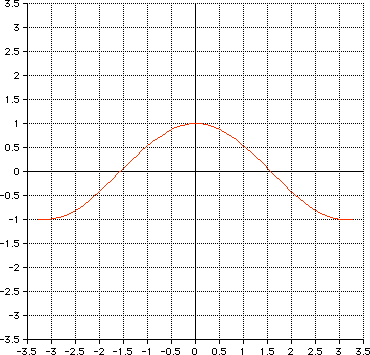Tangent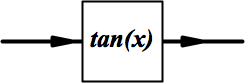Codomain -3.2767 to +3.2767
Graph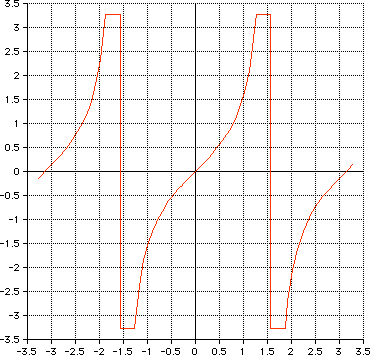ArcSine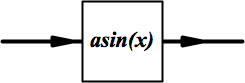Domain -1 to +1
Graph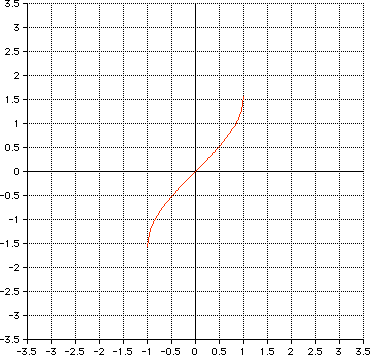ArcCosine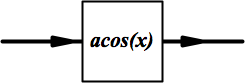Domain -1 to +1
Graph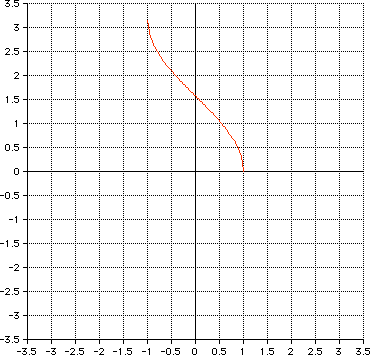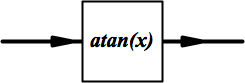Graph